Need a discount on popular programming courses? Find them here. View offers

Don't have an account?

Have you read our submission guidelines?

Go back to

# Top 10 Machine Learning Algorithms for ML Beginners

The utilization and adoption of Machine learning have developed remarkably in the last decade or so. In fact, this age can be called as one of the most revolutionary and significant periods in terms of technology. The impact of machine learning is such that it has begun to lead our lives prevalently.

We are living in a world wherein all things that were once known as manual tasks are getting transformed into automated ones and the reason why this is happening is because of machine learning. It stands as the reason behind the creation of many interactive software applications, robots performing surgeries, computers playing chess, the conversion of large frame computers into PCs, self-driven cars, interactive online learning solutions, amongst many other things.

Technology is consistently evolving at an alarming rate. If we analyze the computing advances made over the recent years, one thing that is easily predictable is the bright future that lies ahead of us.

That being said, the reason why data scientists have been able to address the ever-so complex nature of first-hand problems with technological solutions is by the utilization of special machine learning algorithms that have been developed to solve these problems perfectly well. And to say the least, the outcomes have been remarkable until now.

In this blog, we will list down 10 machine learning algorithms that data scientists must know about. In case you are a data scientist, an aspiring one, or if you’re simply intrigued by machine learning, then knowing about these algorithms will be extremely helpful.

However, before we jump onto learning about the 10 machine learning algorithms, let’s first understand two main types of machine learning techniques. These techniques are the foundation for understanding the ten machine learning algorithms.

There are two main types of machine learning techniques:

### What is Supervised Learning?

The supervised machine learning follows a model that forms predictions dependent on the evidence of uncertainty. To put this in simple terms, a supervised learning algorithm is reliant on a previously noted set of inputs and their relevant outputs. It plays a role in making analytical predictions with the responses to new data.

Data scientists can use supervised learning in case they have already known data for the output that they wish to predict. Furthermore, supervised learning is carried with the usage of classification and regression methods for the enhancement of predictive models.

Classification Technique: This technique is used for predicting rather discrete responses. For example; if you want to find out if an email is authentic or spam, a tumour is malignant or beginning then you can use the classification technique.

The most common applications of this technique include speech recognition, medical imaging, credit scoring, etc. The common machine learning algorithms that are used for performing classification include the k-nearest, discriminant analysis, boosted, bagged decision tree, support vector machine (SVM), neural networks, etc.

Regression Technique: In order to predict the continuous responses such as a change in temperature, fluctuations in the power demand then regression technique is used. The most common applications of this technique include electricity load, algorithmic trading, forecasting, etc.

Data scientists use regression techniques if they are working with a data range or in case the nature of a particular response comes out to be a real number like temperature or time. The machine learning algorithms include linear model, regularization, stepwise regression, bagged decision trees, non-linear model, etc.

### What is Unsupervised Learning?

Unsupervised learning is used when the objective is to find the hidden patterns or any intrinsic structures within the data. It enables the data scientists to draw important inferences from datasets that consist of input data without any labelled responses.

Clustering: The most common unsupervised learning technique is referred to as clustering. This technique is utilized for the purpose of exploratory data analysis to find hidden patterns or groupings in the data. The most common applications of clustering include market research, gene sequence analysis, object recognition, etc.

The commonly used algorithms for performing clustering include k-means and k-medoids, Gaussian mixture models, hierarchical clustering, hidden Markov models, subtractive clustering, etc

Visualization Algorithm: The unsupervised algorithms that affirm the data which is not labelled while showcasing it in the form of an intuitive structure, mostly in 2D or 3D is called a Visualization algorithm. This data set precisely differs in understandable clusters to increase understanding.

Anomaly Detection: The algorithm detection is used to find out the probable anomalies within the data. It can be used for detecting any suspicious credit transactions, differentiating criminals from a set of people, etc.

Rule Data Scientist Follow While Choosing Between Supervised and Unsupervised Machine Learning

• The supervised learning technique is chosen when the data scientists need to train a model for making predictions. For example: predicting the future of a continuous variable such as temperature or a stock price. Additionally, they are also used while doing a classification. For example: finding if an email is from an authentic source or if it is spam.
• Data scientists choose unsupervised learning if there is a need to explore a set of data or training a model for finding a good internal representation like the process of splitting the data into clusters.

Now that we have laid a foundation of the machine learning techniques, let’s jump right into the top 10 machine learning algorithms that all data scientists must know about.

### 1. Linear Regression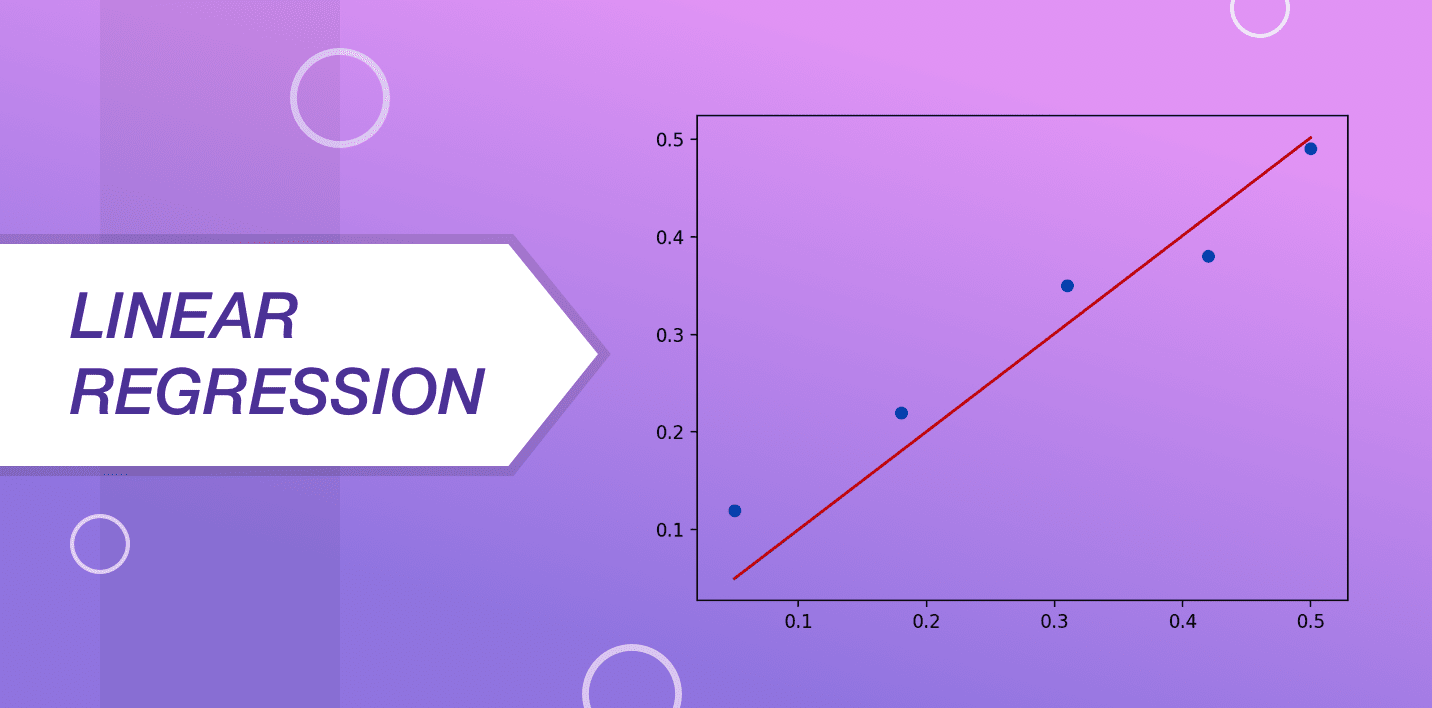Linear regression is known as a highly used and prevalent machine learning algorithm that is utilized by data scientists across the world. In order to understand the functionality of this algorithm, imagine a set of stones that are in the arrangement of ascending weights.

The restriction here is that you cannot weigh these stones by yourself. You will have to guess the weight of these logs by looking at their height and shape while arranging them with a classification that is based on visible parameters. This is precisely what linear regression is all about.

The representation of linear regression is done with an equation defining a line that fits very well into the relationship between the input variables and the output variable. This line is referred to as the regression line and it is mainly shown with an equation:

`Y = a*X + b`

In this equation, “y” is a dependent variable, “a” is the slope, “X” is an independent variable and “b” is the intercept. The goal of linear regression is to find the values of coefficients “a” and “b”.

### 2. Logistic Regression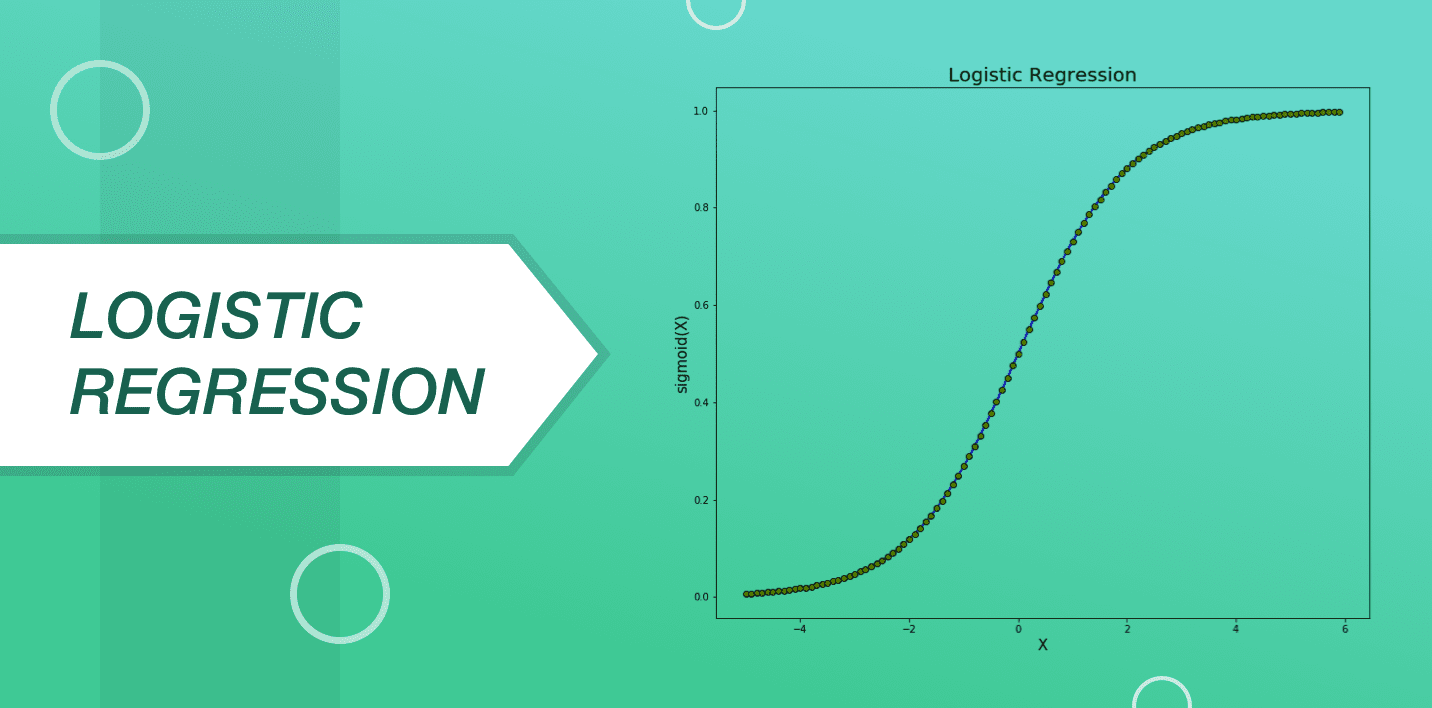This algorithm is derived from the field of statistics. It is considered to be a dependable method for carrying out binary classification problems. The main aim of logistic regression is to discover the values for the coefficients representing all the input variables. Other than linear regression, the common output predicted for logistic regression is changed using a nonlinear function known as a logistic function.

The logistic function is in the shape of a big “S” and is used to change any value ranging from 0 to 1. It is helpful in applying a specific rule to the output of the logistic function for changing values from 0 to 1. Due to the way in which this model is grasped or identifies, the important predictions implemented by the logistic regression can be utilized as the probability of any data that belongs to classes 0 or 1. This can be used to solve problems where more thought needs to be given to a prediction.

Working in a way very similar to linear regression, the logic regression is all the more effective at the time when the attributes that are not linked to the output variable along with the attributes that are the same in nature get removed.

### 3. Decision Tree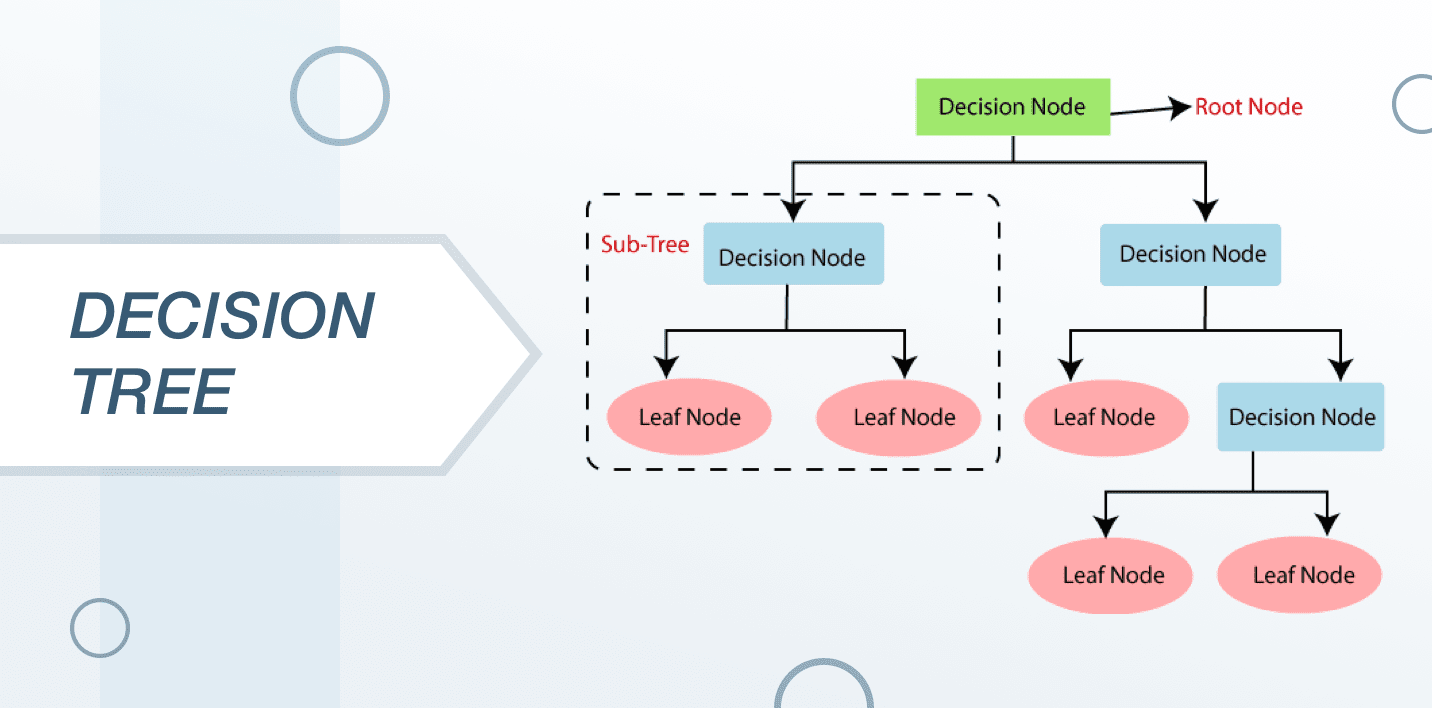The decision tree is one of the most commonly used machine learning algorithms. It is basically a supervised learning algorithm that works very well for classifying problems. It is utilized for classifying both the variables that are both continuous and specific.

Commonly used in statistics and data analytics for the purpose of predictive models, the structure of this algorithm is represented with the help of leaves and branches. The attributes of the objective function are based on the branches of the decision tree, the values of the objective function are recorded in the leaves and the remaining nodes contain attributes for which the cases differ.

In order to classify a new case, the data scientists are supposed to go down the lead in order to give the appropriate value. The objective is to create a model that can predict the value of the target variable which is dependent on many input variables.

### 4. Support Vector Machines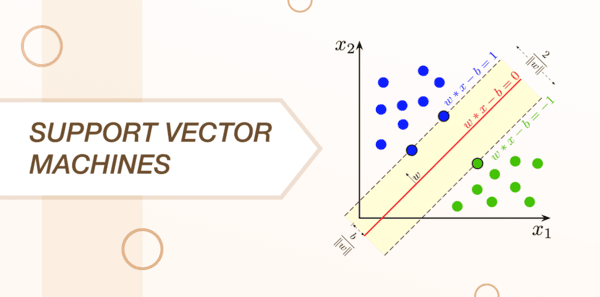A linear model which works very much on the lines of both linear and logistic regression is called as the support vector machine. It is more like a method of classification in which the data scientists plot raw data with points in the n-dimensional space; where n is known as the number of features.

Every feature has a value which then gets tied up to a particular coordinate that makes the process of classifying data very simple. The data is then split up and plotted in a detailed manner on a graph with lines called classifiers.

The SVM algorithm is commonly used to define an optimal hyperplane with an optimized margin, map data to a high dimensional space so that it becomes easier to classify with linear decision surfaces and reformulate problems so that the data is mapped implicitly into space.

### 5. Naive Bayes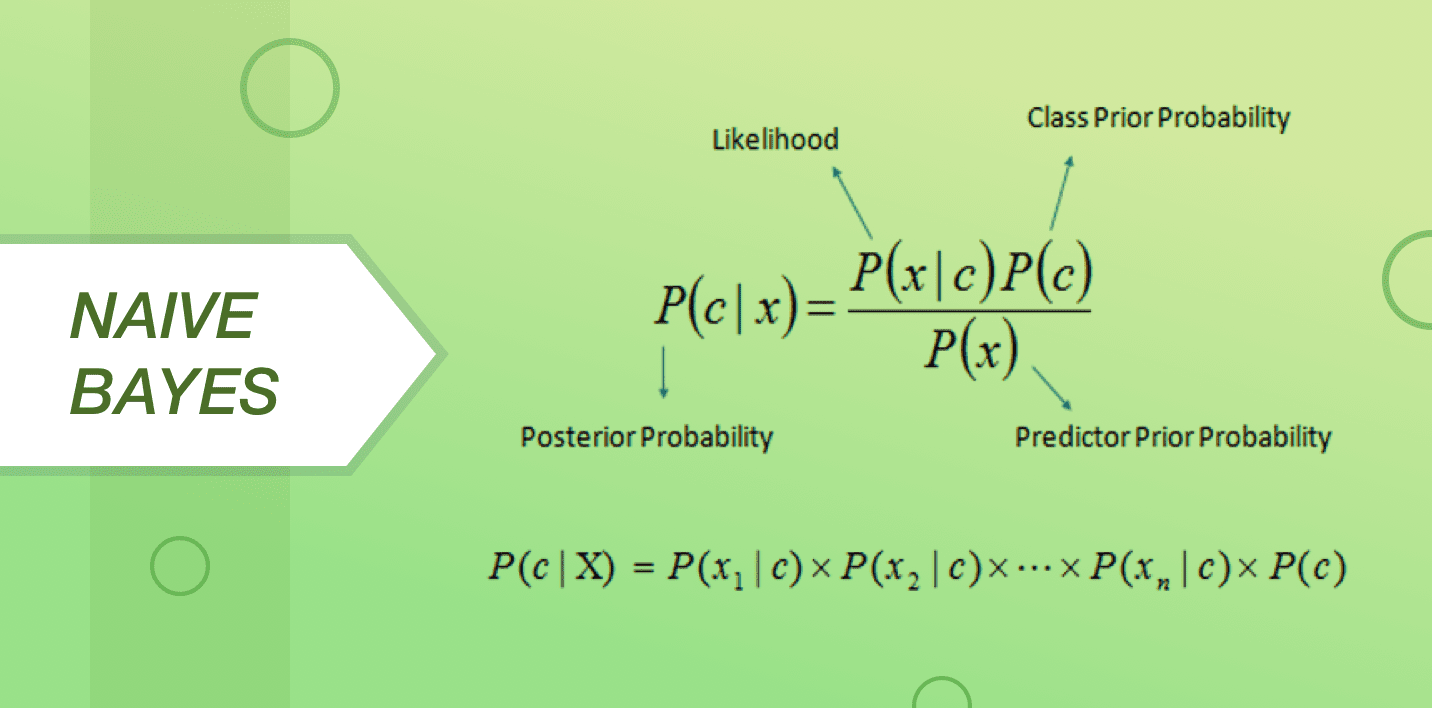Naive Bayes is popularly known as an easy to use but an impactful machine learning algorithm that is utilized for the purpose of predictive modelling. It is dependent on a model that majorly includes two types of probabilities that are calculated straight up by the inputs of training data.

The probability of every class is considered as the first and the conditional probability for every class given x value is considered second. Upon the completion of the calculation, the probability model is used for making predictions on new data with the help of the Bayes Theorem. In case the data is based on real values, it is more than common to consider it as a Gaussian distribution so that the probabilities can be quickly derived.

Naive Bayes has the word naive due to the fact that it assumes each input variable to be independent which can be a strong and unrealistic assumption for the real data. Regardless, this technique is extremely impactful when it comes to a wide range of difficult problems.

### 6. k-NN (k-Nearest Neighbours)The k-NN algorithm can be used for the classification as well as the regression problems. It is used very commonly by the data scientists for solving the data classification problems. It is a pretty easy algorithm that is used for storing the available cases while also labelling any discovered cases or taking a relevant vote of k-neighbours.

After that, the case is assigned to the class with which it matches commonly. A distance function is commonly used for performing this measurement. KNN requires a major part of the memory for the purpose of storing the data, but it only carries out a calculation at the time when a prediction is required. It is possible to update training partly with time just for the sake of maintaining precise predictions.

### 7. K-Means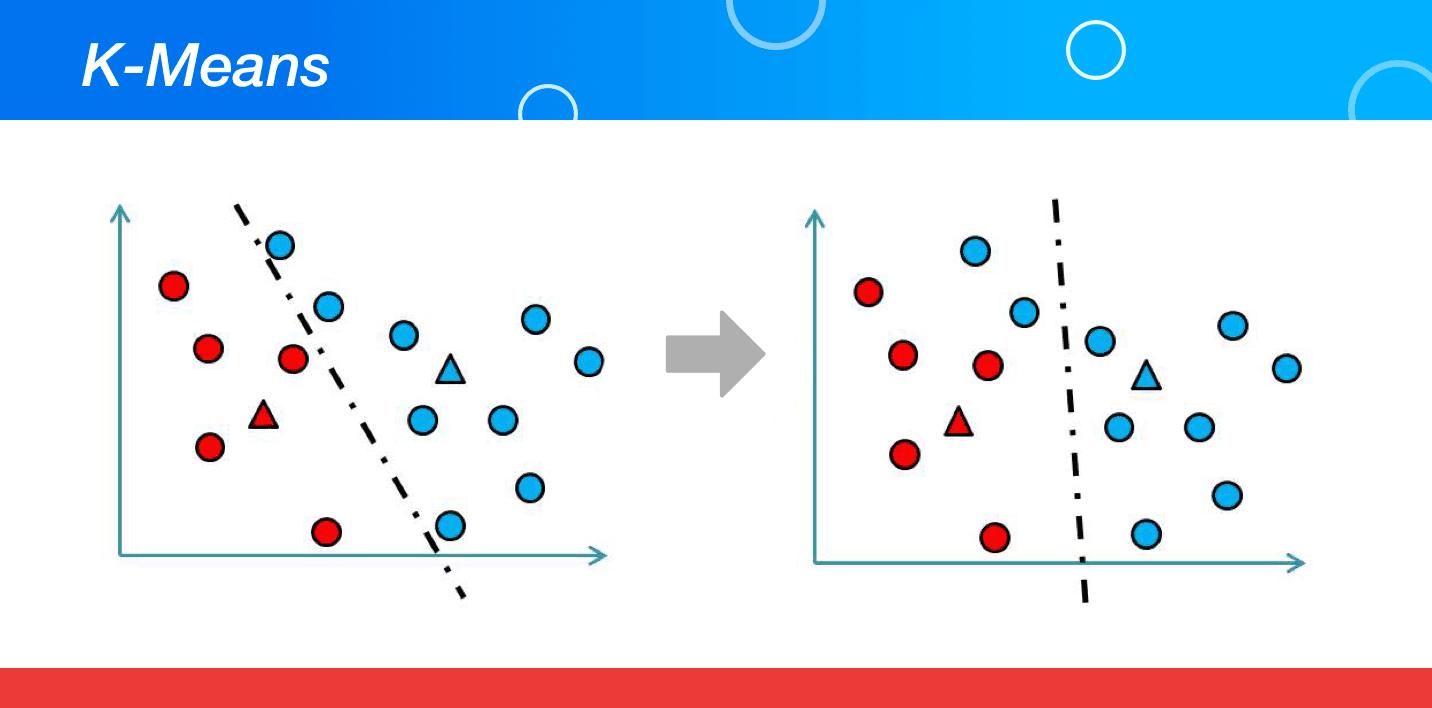An unsupervised algorithm that is mainly used for solving clustering problems is called K-Means. The data sets in this algorithm are classified into a number of data sets following a particular number of clusters. This is carried out in a manner that all the data points falling within a cluster turn homogeneous or heterogeneous in comparison to the data that falls in other clusters.

K-Means form clusters by picking a “k” number of points called centroids for every cluster. All the data points create a cluster with the nearest centres of the K clusters. It then creates new centroids dependent on the existing cluster members. With the help of new centres, the nearest distance for every data point gets discovered. This process keeps occurring till the time centroids reach a point of no change.

### 8. Random Forest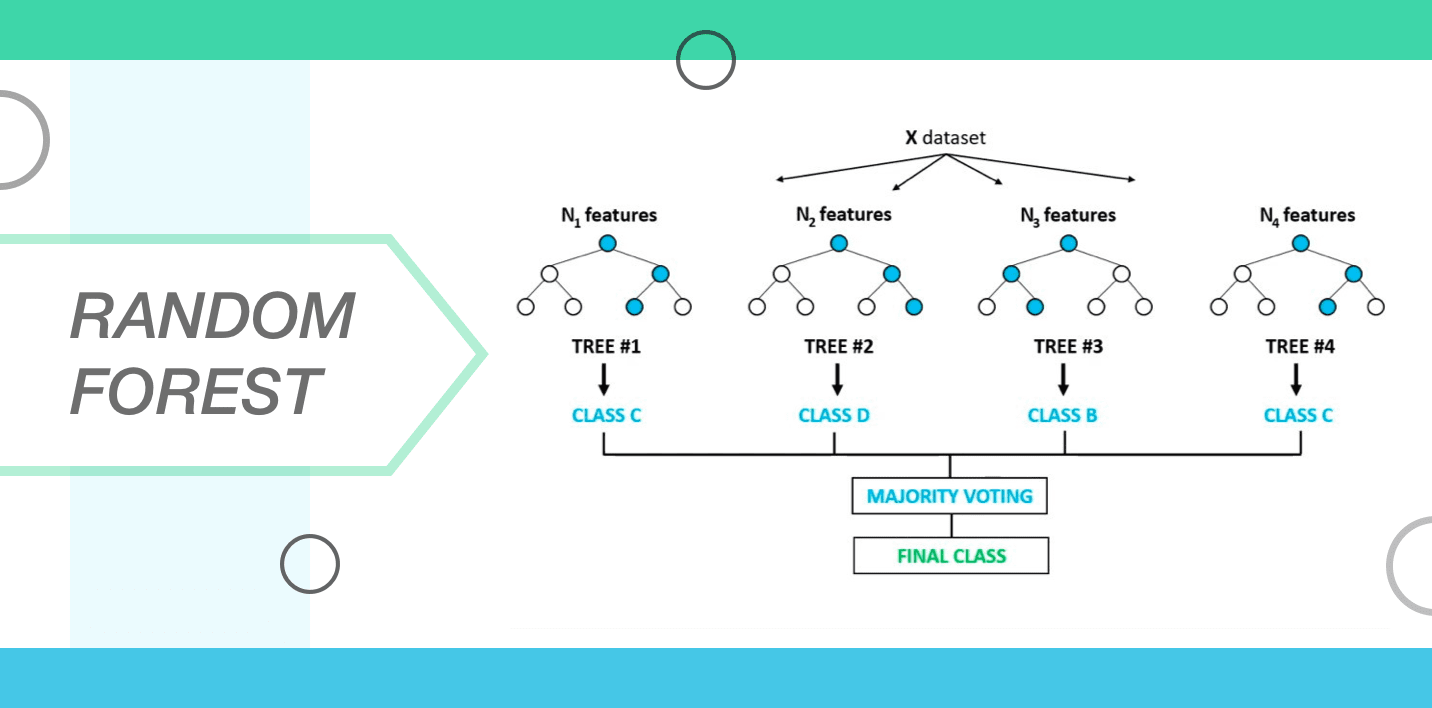A group of decision trees is commonly referred to as a random forest. In order to classify a new object which is reliant on its attributes, all the trees are classified and they vote for the class. Following this, the forest chooses the classification that has the maximum number of votes as compared to all other trees in the forest. All the trees get planted and they grow as mentioned below.

• The number of cases in the training set is taken as “N”. A sample of “N” cases is then taken at random and this sample is used as a training set for growing the tree.
• At the time when the input variables are M then a number m<M gets labelled so that at every node, the m variable gets selected at random out of M variables and the best split on m can be used for splitting the mode. The m value that gets derived is known as a constant throughout the whole process.
• Every tree is grown to the extent that there is no pruning.

### 9. Dimensionality Reduction Algorithms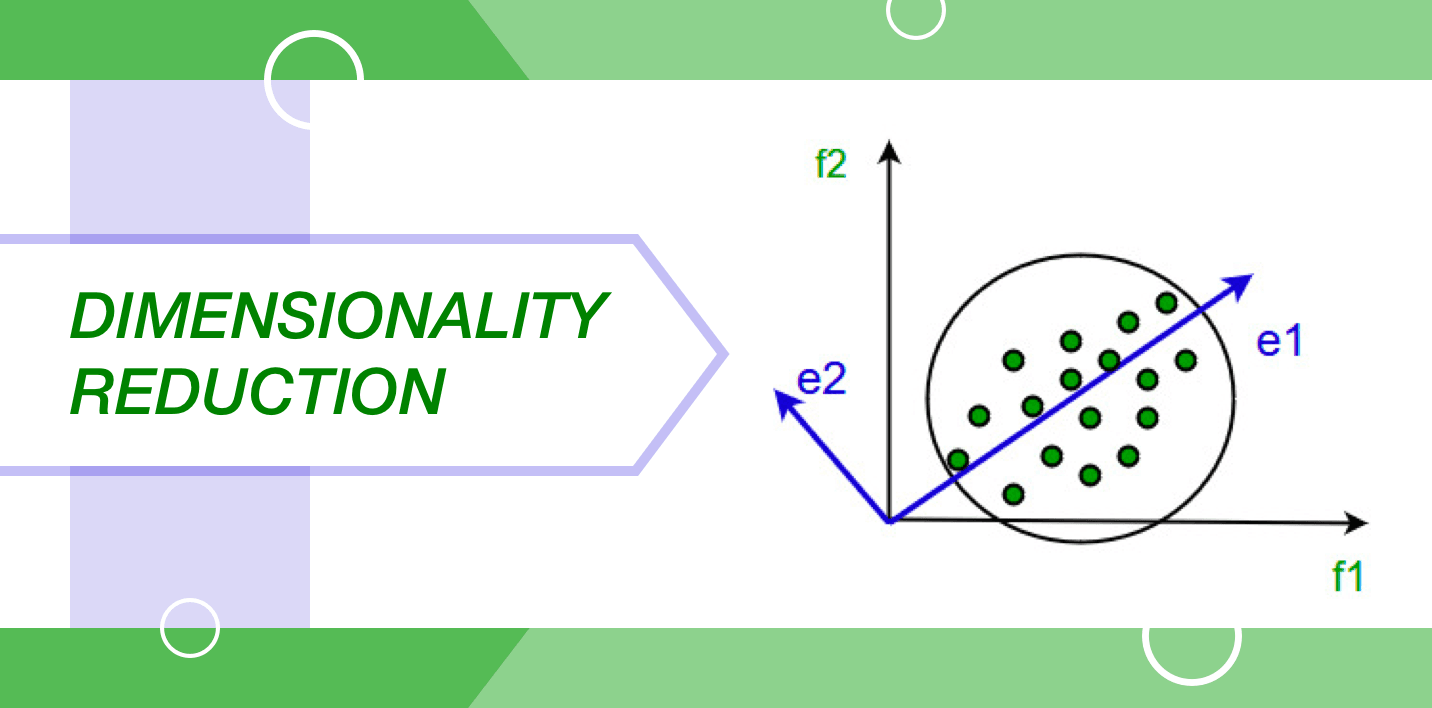In the present world, there is a huge amount of data that is stored and analyzed by organizations of all kinds. It is the responsibility of the data scientist to ensure that this raw data stays protected. Due to the fact that it contains huge information, the most important limitation is to identify relevant patterns and variables.

The dimensionality reduction algorithms like Factor Analysis, Random Forest, Missing Value Ratio, Decision Tree, etc. can assist in finding the important details.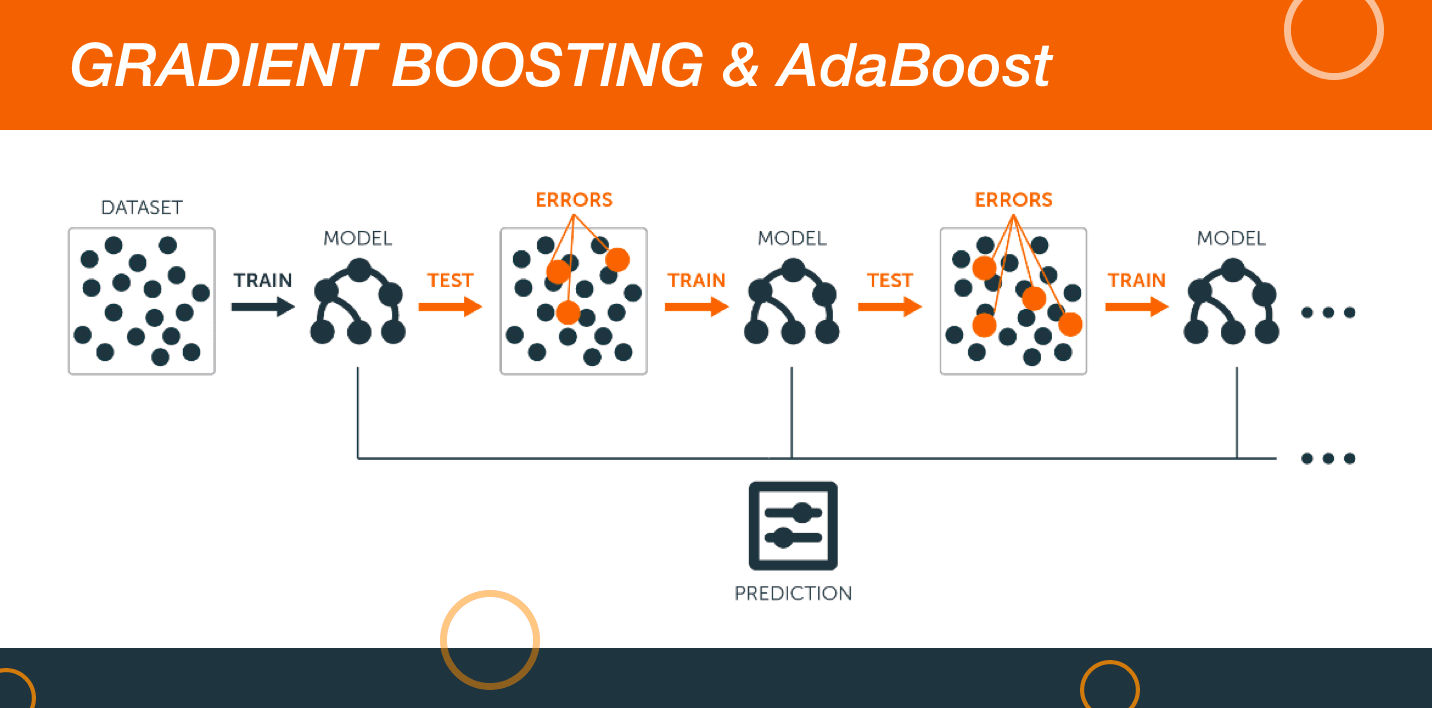The two algorithms utilize a large amount of data for managing predictions with precision. More or less, boosting is like an ensemble learning algorithm that includes the predictive learning power of various base estimators for enhancing robustness.

In simple terms, it brings together the weak predictors for the purpose of creating a rather strong predictor. The boosting algorithms have a reputation of working really well in the data science competitions. They are considered as highly preferred machine learning algorithms. Data scientists mainly use them with Python and R Codes for achieving accurate outcomes.

## Conclusion

Above mentioned is the list of ten machine algorithms that all data scientists must know about. A very common decision made by beginners in the field of data science is choosing the right data science algorithm to use. The correct choice for this factor depends entirely on some important highlights that include the size, the quality level, data’s nature, computational time at hand, task priorities, and the aim for utilizing the data.

Therefore, regardless of the fact if you are new or experienced in the field of data science, choose any one of the algorithms mentioned above to get maximum results.

Which ML algorithms would you choose and for which scenarios? Let us know!

STAY IN LOOP TO BE AT THE TOP

#### Welcome to the club and Thank you for subscribing!

By Simran Kaur Arora

Simran works at Hackr as a technical writer. The graduate in MS Computer Science from the well known CS hub, aka Silicon Valley, is also an editor of the website. She enjoys writing about any tech topic, including programming, algorithms, cloud, data science, and AI. Traveling, sketching, and gardening are the hobbies that interest her.

View all post by the author

Disclosure: Hackr.io is supported by its audience. When you purchase through links on our site, we may earn an affiliate commission.